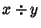## Division

Taking the Ratioof two numbersand, also written. Here,is called the Divisor. The symbol ``/'' is called a Solidus (or Diagonal), and the symbol ``'' is called the Obelus. Division in which the fractional (remainder) is discarded is called Integer Division, and is sometimes denoted using a backslash,.

See also Addition, Divide, Integer Division, Long Division, Multiplication, Obelus, Odds, Ratio, Skeleton Division, Solidus, Subtraction, Trial Division